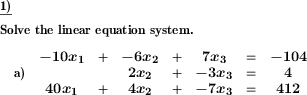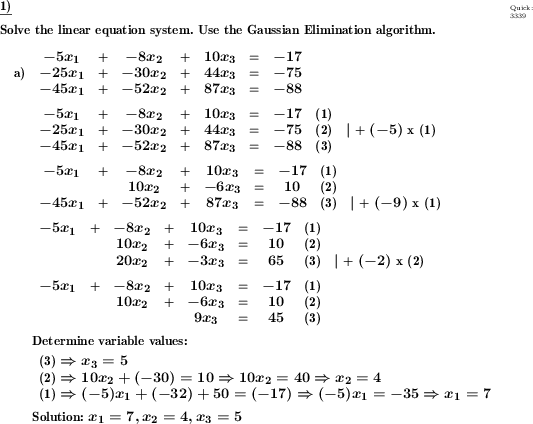Custom math worksheets at your fingertipsDetails for problem "Linear equation system - Gaussian algorithm"

Quickname: 3339

Junior High School, High School, Senior High School.

Summary

A system of linear equations is to be solved with the Gaussian elimination method.

ExamplesDescription

A system of linear equations with two to four unknowns is to be solved. According to the number of unknowns a number of linear equations are given.

The names of the unknowns can be chosen from the following naming schemes : x1 to x4, a,b,c,d... or w,x,y,z.

The given system will always be solvable unambiguously.

The following types of problems are available:

- Gaussian elimination method with integers

The solution can optionally be output by

• simple indication of the solution
• output of the triangular form of the equation system, followed by the derivation of the unknowns by incremental substitution.
• the output of the solution steps with to the triangle form, then followed by the derivation of the unknowns by incremental substitution.

If desired, a reference to the application of the Gauss method can be given with the task definition. The number of unknowns and thus the size of the task is selectable. The number of problems can also be set.

Download free printable worksheets for this math problem here. The worksheet contains the problems only, the solution sheet includes the answers. Just click on the respective link.

•Worksheet 1Solution sheet with answers
•Worksheet 2Solution sheet with answers
•Worksheet 3Solution sheet with answers

If you can not see the solution sheets for download, they may be filtered out by an ad blocker that you may have installed. If this is the case, please allow ads for this page and reload the page. The solution sheets will then reappear.

• Do these sample worksheets do not really fit?
• Do you need more math worksheets, with a different level of difficulty?
• Would you like to combine different problems on a worksheet and adjust them to your needs?
• As a teacher, you can put together your own worksheets using the automatically generated math problems provided.
With a free initial credit, you can start creating your own math worksheets in a few minutes.

You can try it for free! Register here, to create custom worksheets now!

Customization options for this problem

Parameter
Possible values
Number of problems
1, 2
Solution verbosity
Solutions only, Triangular form, Steps to solve
Type
Gauss, whole nmbrs
Size
2, 3, 4
Variable names
x1,x2,x3,x4, w,x,y,z, a,b,c,d
Mention Gauss Algorithm
Yes, No

Similar problems

Remark
Description
with only one unknown
Solve a linear equation step-by-step by performing equivalent transformations.Deutsche Version dieser Aufgabe
These informational pages with samples describe math problems that can be combined on custom math worksheets with solutions for home and K-12 school use.
Deutsche Seiten
×